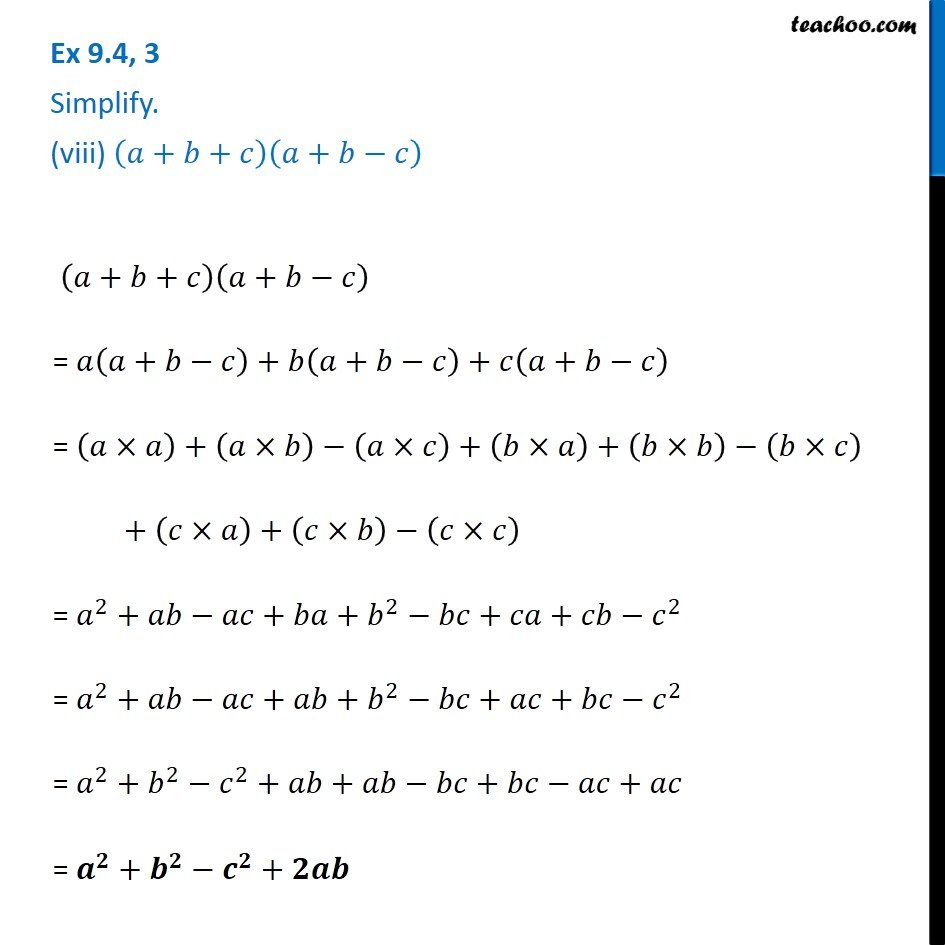Subscribe to our Youtube Channel - https://you.tube/teachoo

1. Chapter 9 Class 8 Algebraic Expressions and Identities
2. Concept wise
3. Multiplication of Polynoimals by Polynomials

Transcript

Ex 9.4, 3 Simplify. (viii) (𝑎+𝑏+𝑐)(𝑎+𝑏−𝑐) (𝑎+𝑏+𝑐)(𝑎+𝑏−𝑐) = 𝑎(𝑎+𝑏−𝑐)+𝑏(𝑎+𝑏−𝑐)+𝑐(𝑎+𝑏−𝑐) = (𝑎×𝑎)+(𝑎×𝑏)−(𝑎×𝑐)+(𝑏×𝑎)+(𝑏×𝑏)−(𝑏×𝑐) + (𝑐×𝑎)+(𝑐×𝑏)−(𝑐×𝑐) = 𝑎^2+𝑎𝑏−𝑎𝑐+𝑏𝑎+𝑏^2−𝑏𝑐+𝑐𝑎+𝑐𝑏−𝑐^2 = 𝑎^2+𝑎𝑏−𝑎𝑐+𝑎𝑏+𝑏^2−𝑏𝑐+𝑎𝑐+𝑏𝑐−𝑐^2 = 𝑎^2+𝑏^2−𝑐^2+𝑎𝑏+𝑎𝑏−𝑏𝑐+𝑏𝑐−𝑎𝑐+𝑎𝑐 = 𝒂^𝟐+𝒃^𝟐−𝒄^𝟐+𝟐𝒂𝒃

Multiplication of Polynoimals by Polynomials

About the AuthorDavneet Singh
Davneet Singh is a graduate from Indian Institute of Technology, Kanpur. He has been teaching from the past 9 years. He provides courses for Maths and Science at Teachoo.